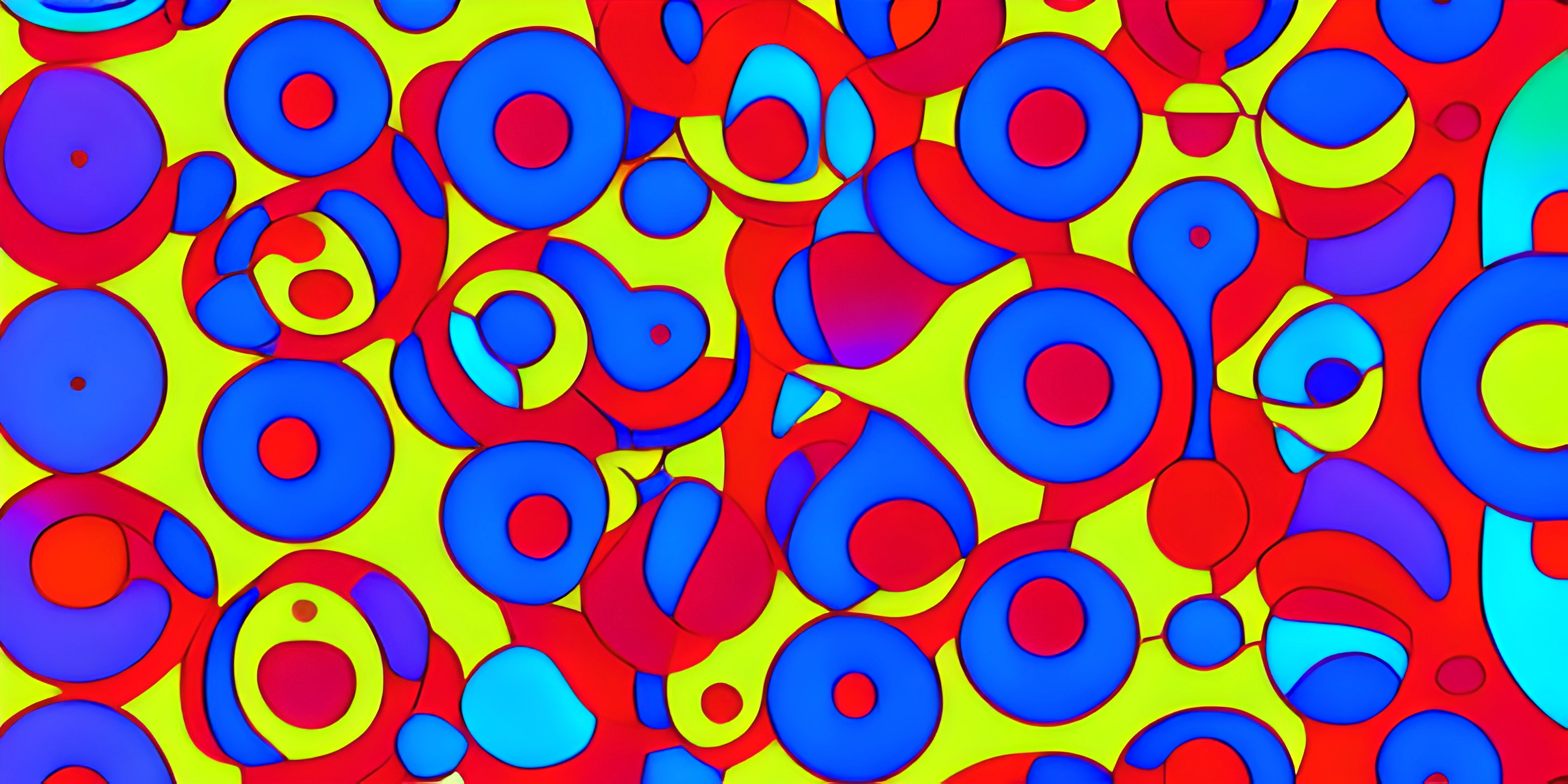# Disjoint Set Union: Optimizing Union and Find OperationsNote: this page has been created with the use of AI. Please take caution, and note that the content of this page does not necessarily reflect the opinion of Cratecode.

Optimizing union and find operations is a common challenge when working with data structures. One solution to this challenge is the disjoint set union data structure. The disjoint set union, also known as union-find, is a handy technique that efficiently handles sets with a focus on merging them and checking if elements belong to the same set.

## Understanding the Disjoint Set Union

A disjoint set union is a collection of non-overlapping sets, where each element belongs to exactly one set. The purpose of this data structure is to perform two primary operations: union and find.

### Union Operation

The union operation is used to merge two sets into one. If we have two sets `A` and `B`, the union operation will combine all elements of `A` and `B` into a new set, removing the original sets from the collection.

### Find Operation

The find operation is used to determine which set an element belongs to. Given an element `x`, the find operation will return the unique identifier of the set containing `x`.

## Disjoint Set Union with Path Compression and Union by Rank

Two optimizations that are widely used with disjoint set unions are path compression and union by rank. These optimizations help to keep the data structure's tree shallow, reducing the time complexity of union and find operations.

### Path Compression

Path compression is an optimization that, during a find operation, compresses the path from the element being searched to the root element. This compression is done by updating each node in the path to point directly to the root. As a result, the height of the tree is reduced, speeding up future find operations.

### Union by Rank

Union by rank is an optimization that, during a union operation, helps to keep the tree shallow by attaching the shorter tree to the root of the taller tree. This approach minimizes the height of the resulting tree, allowing for faster find operations.

## Implementation Example

Here's an example implementation of a disjoint set union with path compression and union by rank using Python:

``````class DisjointSetUnion:
def __init__(self, size):
self.parent = list(range(size))
self.rank =  * size

def find(self, x):
if self.parent[x] != x:
self.parent[x] = self.find(self.parent[x])
return self.parent[x]

def union(self, x, y):
root_x = self.find(x)
root_y = self.find(y)

if root_x == root_y:
return

if self.rank[root_x] > self.rank[root_y]:
self.parent[root_y] = root_x
else:
self.parent[root_x] = root_y
if self.rank[root_x] == self.rank[root_y]:
self.rank[root_y] += 1``````

In this implementation, the `DisjointSetUnion` class has a `parent` list to store the parent of each element and a `rank` list to store the rank of each element. The `find` method implements path compression, and the `union` method implements union by rank.

The disjoint set union data structure is a powerful technique for optimizing union and find operations in programming. By understanding and implementing this data structure with path compression and union by rank, you can efficiently handle sets and their operations in your code.

## FAQ

### What is a disjoint set union?

A disjoint set union, also known as a union-find data structure, is a data structure that efficiently keeps track of a partition of a set into disjoint subsets. It supports two main operations: union and find. The union operation combines two subsets into a single subset, while the find operation determines the representative of a given element's subset.

### How does the disjoint set union data structure work?

The disjoint set union data structure represents each subset using a tree, where each node stores an element and a reference to its parent. The root node of the tree is the representative of the subset. The union operation merges two subsets by attaching the root of one tree to the root of the other. The find operation retrieves the representative of a subset by traversing up the tree until the root node is reached.

### What are the main optimizations for union and find operations?

There are two main optimizations that can be applied to the disjoint set union data structure to improve the efficiency of union and find operations:

1. Union by rank: When merging two subsets, attach the smaller tree to the root of the larger tree. This helps keep the height of the trees smaller, resulting in faster find operations.
2. Path compression: During a find operation, flatten the tree by making each visited node point directly to the root. This speeds up future find operations on the same elements. Here's an example of implementing these optimizations in Python:
``````class DisjointSetUnion:
def __init__(self, size):
self.parent = list(range(size))
self.rank =  * size
def find(self, x):
if self.parent[x] != x:
self.parent[x] = self.find(self.parent[x])
return self.parent[x]
def union(self, x, y):
root_x = self.find(x)
root_y = self.find(y)
if root_x == root_y:
return False
if self.rank[root_x] > self.rank[root_y]:
self.parent[root_y] = root_x
else:
self.parent[root_x] = root_y
if self.rank[root_x] == self.rank[root_y]:
self.rank[root_y] += 1
return True``````

### When should I use a disjoint set union data structure?

A disjoint set union is particularly useful in situations where you need to efficiently manage a collection of disjoint sets and perform union and find operations. Some common use cases include solving problems related to graph theory, such as finding connected components, determining cycle presence in an undirected graph, and implementing Kruskal's algorithm for finding minimum spanning trees.

### Can I use disjoint set union with other data structures?

Yes, disjoint set union can be combined with other data structures such as arrays, lists, or dictionaries to support more complex data manipulation and storage requirements. The key idea is to adapt the union and find operations according to the chosen data structure while maintaining the optimizations for improved efficiency.